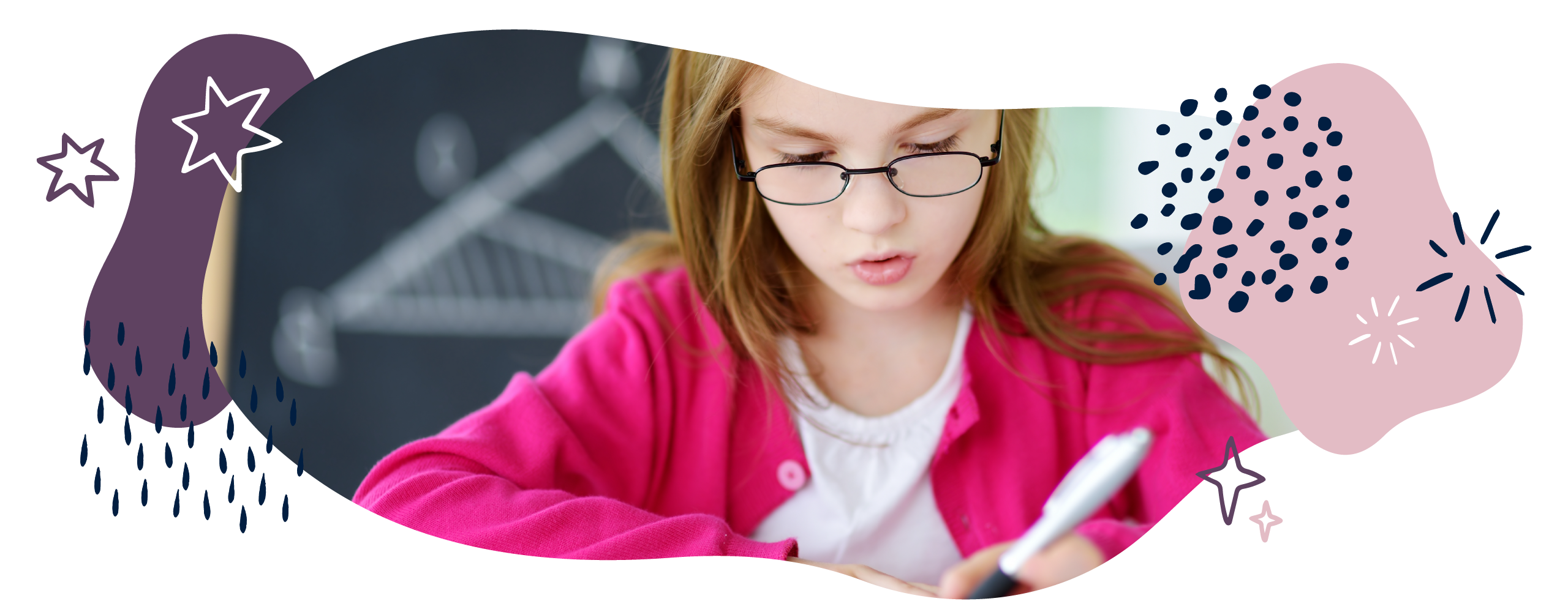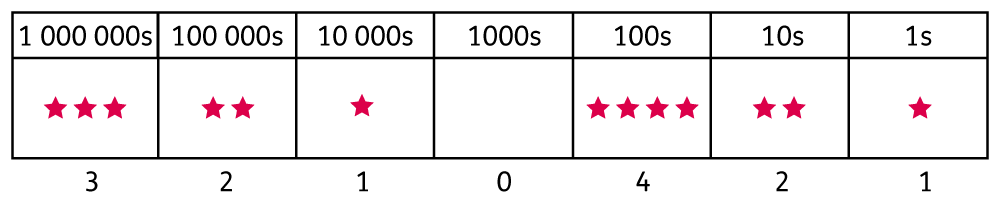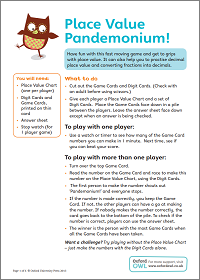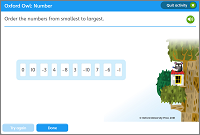We use cookies to enhance your experience on our website. By continuing to use our website, you are agreeing to our use of cookies. You can change your cookie settings at any time. Find out more# Number & place value in Year 5 (age 9–10)

In Year 5, your child will be reading, writing, and comparing numbers up to 1,000,000. They will round large numbers, interpret negative numbers in lots of contexts, and learn Roman numerals up to 1000 (M).

The key words for this section are number and place value.

## What your child will learn

Take a look at the National Curriculum expectations for number and place value in Year 5 (ages 9–10):

#### Read, write, and compare numbers up to at least 1,000,000

Place value is the basis of our number system and allows us to represent all our numbers with just the digits 0–9. Your child will understand that the position of the digit in a number tells us the value of the digit.

Your child will know the value of each digit in numbers up to at least a million. They will be able to order and compare numbers using their understanding of place value.Note that the children will use the vocabulary ones and not units when talking about place value.

#### Count in powers of 10 from any number up to 1,000,000

Your child will use the language of ‘powers of’, which means the number of times a number is multiplied by itself. So 10 to the power of 4 (written as 10⁴) would be 10 × 10 × 10 × 10 = 10,000.

Your child will be expected to count forwards and backwards in powers of 10 from any number up to a million.

Count forwards in steps of 10² (100) from 25,760: 25,860, 25,960, 26,060, and so on.

Count backwards in steps of 10³ (1000) from 8151: 7151, 6151, 5151, and so on.

#### Interpret and use negative numbers

Your child will interpret negative numbers in real-world contexts. For example, they might learn about negative numbers using temperature or money.

Your child will also count forwards and backwards with positive and negative whole numbers, including counting through 0. For example:

Count backwards from 6 to -3: 6, 5, 4, 3, 2, 1, 0, -1, -2, -3.

#### Round numbers to the nearest 10, 100, 1000, 10,000, and 100,000

Your child will round numbers up to a million up or down to the nearest 10, 100, 1000, 10,000 and 100,000.

537,129 rounded to the nearest 1000: 537,000.

145,674 rounded to the nearest 10,000: 150,000.

832,541 rounded to the nearest 100,000: 800,000.

#### Solve increasingly complex number problems

Children will solve problems involving:

• Counting
• Ordering
• Comparing
• Rounding
• Negative numbers
• Roman numerals.

Their knowledge of place value will be very useful for this. They will use physical objects, drawings, diagrams, and mathematical symbols to visualise problems.

#### Read Roman numerals to 1000 (M)

Your child will be able to read Roman numerals from 1 (I) to 1000 (M). They should be able to recognise years written in Roman numerals.

1 = I

5 = V

10 = X

50 = L

100 = C

500 = D

1000 = M

1995 = MCMXCV

2019 = MMXIX

## How to help at home

There are lots of ways you can help your child to understand number and place value. Here are just a few ideas:

### 1. Use place value charts

Place value charts can be a great way to help your child represent numbers and understand how the number system works. These charts will help your child to read, write, and compare numbers, as well as to understand how zero is used as a placeholder.

For example, 210,421 could be represented as follows:### 2. Use place value knowledge

When comparing numbers up to 1,000,000, your child should look at the digit with the largest value first. For example, if your child is comparing the numbers 232,765 and 233,276, they would first need to look at the digit with the largest value. In this example, as the hundred thousands and ten thousands digits are the same, we need to look at the thousands digit:

232,765 has 2 thousands, and 233,276 has 3 thousands, so 232,765 is less than 233,276.

Encourage your child to use their knowledge of number and place value to identify patterns when calculating. For example, if you know 4 + 3 = 7, then you also know 0.4 + 0.3 = 0.7. This is because each of the numbers in the calculation is now ten times smaller, so the total will be ten times smaller.

Give your child a simple addition calculation using one-digit numbers such as 5 + 4 = 9. How many related calculations can they record using this fact? For example:

15 + 4 = 19

5 + 14 = 19

50 + 40 = 90

500 + 400 = 900

0.5 + 0.4 = 0.9

+  =

… and so on.

### Activity: Place value pandemonium### 3. Explore negative numbers

Chatting about the numbers we see around us will help your child use numbers in all parts of their daily lives. Point out negative numbers in the real world, such as temperatures on the weather forecast. Vertical number lines, such as those seen on thermometers, can help your child grasp the concept of negative numbers.

To help support your child’s understanding of negative numbers, have a go at our negative number ordering game:

### Activity: Negative numbersOrder the numbers from smallest to largest.

### 4. Make estimates

Being able to make accurate estimates is a valuable skill we use in everyday life. When calculating, encourage your child to use their rounding skills to estimate the answer before calculating precisely. This will help them to check if their answer seems reasonable.

If you are out shopping and you have picked up a few items, ask your child to estimate the total cost of the items. They will need to round the cost of each item to find the estimated total. For example, if you bought items costing £3.82, 82p, £4.10, and £2.45, your child could round each of these items to the nearest pound:

£3.82 to £4

82p to £1

£4.10 to £4

£2.45 to £2

Your child could then add the rounded amounts of £4, £1, £4, and £2 to find the estimated total of £11. When they calculate precisely and come to £11.19, they can feel fairly confident that their calculation was correct.

### 5. Play Roman numerals bingo

Your child will be expected to be able to read Roman numerals from 1 to 1000. Point out Roman numerals in the modern world. For example, Roman numerals are used on older buildings to show when they were built, or at the end of a film to show the year it was made.

Why not play Roman numeral bingo with your child? Create bingo boards using pieces of A4 paper. Choose and write down six different Roman numerals between 1 and 100 on each piece of paper (one piece of paper per player). One player chooses a number between 1 and 100 and announces it. If a player has that number shown in Roman numerals, they can cover that number. The first player to cover all numbers is the winner.

Here are the Roman numerals up to 1000, with some examples of years written in Roman numerals:

1 = I

5 = V

10 = X

50 = L

100 = C

500 = D

1000 = M

1995 = MCMXCV

2019 = MMXIX